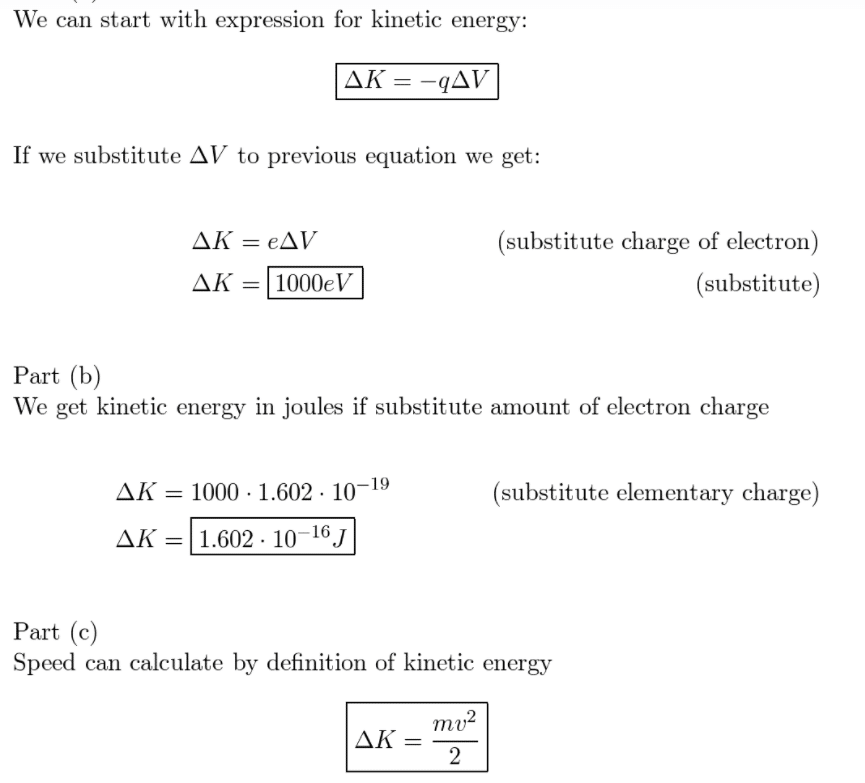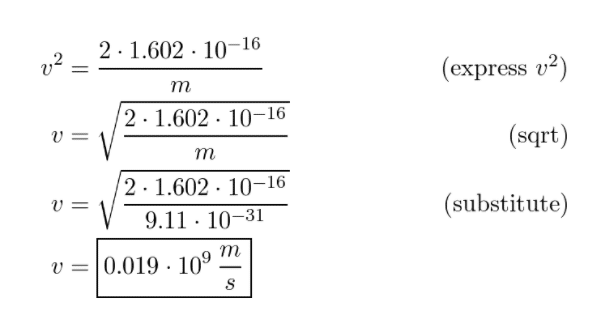# An Electron Has Been Accelerated From Rest Through A Potential Difference Of 1000 V

We thoroughly check each answer to a question to provide you with the most correct answers. Found a mistake? Let us know about it through the REPORT button at the bottom of the page.

An electron has been accelerated from rest through a potential difference of 1000 V.

a. What is its kinetic energy, in electron volts?

b. What is its kinetic energy, in joules?

c. What is its speed?

Answer: v = 0.019 * 10^9 m/s.

## Explanation